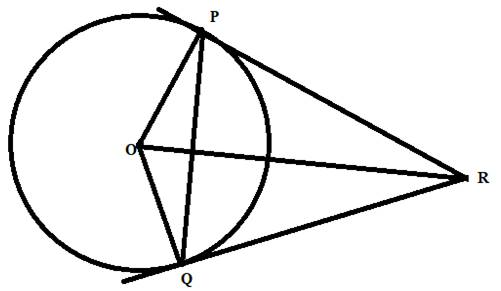# The ratio of area A ( θ ) and B ( θ ) . lim θ → 0 A ( θ ) B ( θ )### Single Variable Calculus: Concepts...

4th Edition
James Stewart
Publisher: Cengage Learning
ISBN: 9781337687805### Single Variable Calculus: Concepts...

4th Edition
James Stewart
Publisher: Cengage Learning
ISBN: 9781337687805

#### Solutions

Chapter 4, Problem 8P
To determine

## The ratio of area A(θ) and B(θ) .  limθ→0A(θ)B(θ)

Expert Solution

### Explanation of Solution

Given information:

An arc PC of a circle subtends a central angle θ .

A(θ) is the area between the cord PQ and the arc PQ.

B(θ) is the area between the tangents PR and QR and the arc.

Calculations:

According to the given information we draw the figure.Here PQ is the cord of the circle and PR and QR are tangents to the circle.

Angle POQ=θ .

Now since PR and QR are tangents to the circle, Therefore,

OPR=OQR=90°.and,PR=QR

Now area of the segment A(θ) is

A(θ)=12r2(θsinθ)

Now Area of B(θ) is,

B(θ)=Area of quadrelateral OPRQ  Area of sector.Area of quadrelateral OPRQ =Area of tringle OPR+Area of triangle OQR.Now,Area of tringle OPR=Area of triangle OQR.Area of tringle OPR=12OP×PR.PR=rtanθ2                     Where r is the radius of the circle.Therefore,Area of tringle OPR=12r×rtanθ2 =12r2tanθ2 Area of quadrelateral OPRQ =2×12r2tanθ2 =r2tanθ2 Area of sector=θ360πr2.Therefore,B(θ)=Area of quadrelateral OPRQ  Area of sector.B(θ)=r2tanθ2θ360πr2. B(θ)=r2tanθ212θr2.

Now ratio of the area,

A(θ)B(θ)=12r2(θsinθ)r2tanθ212θr2A(θ)B(θ)=12(θsinθ)tanθ212θA(θ)B(θ)=(θsinθ)2(tanθ212θ)A(θ)B(θ)=(θsinθ)tanθ2θ

### Have a homework question?

Subscribe to bartleby learn! Ask subject matter experts 30 homework questions each month. Plus, you’ll have access to millions of step-by-step textbook answers!